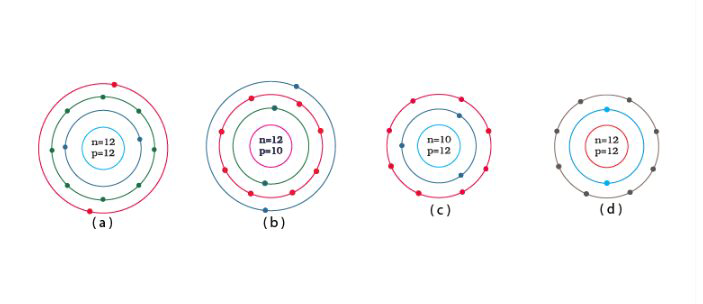# NCERT Exemplar Solutions Class 9 Science Solutions for Structure of the Atom - Exercise in Chapter 4 - Structure of the Atom

Identify the Mg2+ ion from Fig.4.1 where n and p represent the number of neutrons and protons respectively.Explanation:

Number of protons in Mg atom = 2+ 8 + 2 = 12

Number of neutrons in Mg atom = 24 -12 = 12[as mass number of Mg atom = 24 and number of neutrons = mass number – number of protons]

Therefore, option (d) is the correct answer

Related Questions
Exercises

Lido

Courses

Teachers

Book a Demo with us

Syllabus

Maths
CBSE
Maths
ICSE
Science
CBSE

Science
ICSE
English
CBSE
English
ICSE
Coding

Terms & Policies

Selina Question Bank

Maths
Physics
Biology

Allied Question Bank

Chemistry
Connect with us on social media!